## Cipher## Dec 23, 2016

### Adolf Hitler - SS - Nazi Germany

Starting with Paul von Hidenburg who was President of Germany before HitlerHe was a German Military Officer and 2nd President of Germany
Military = 4+9+3+9+2+1+9+7 = 44, Officer = 6+6+6+9+3+5+9 = 44

He died at the age of 86 from 'lung cancer'Died on August Second, 1934 which is the day when Hitler became Fuhrer of Germany
'August' = 1+3+7+3+1+2 = 17 Kill = 2+9+3+3 = 17 - E. Reduction
'Second' = 10+5+3+6+5+4 = 33 with the S exception

8/2/1934 - 8+2+1+9+3+4 = 27 (died in 'Neudeck' = 5+5+3+4+5+3+1 = 27)
8/2/34 - 8+2+34 - 44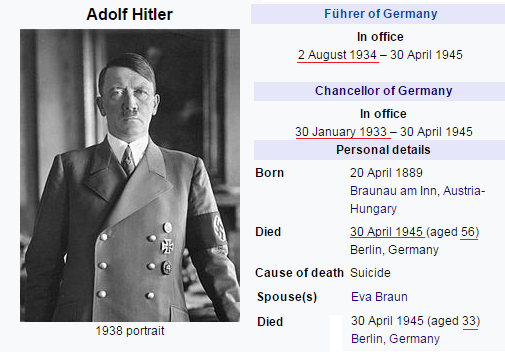Born on April 20th in 1889 which is 110th day in the yearPresident = 7+9+5+10+9+4+5+5+2 = 56 with the S exception just like Adolf Hitler

The date of his birth can also be written like this - 4/20/89 - 4+20+89 = 113
He became Chancellor of Germany on 1/30/33 = 64 (64th prime number is 113)

1/30/1933 - 1+30+1+9+3+3 = 47 (Authority = 47, Foundation = 47, Obey = 47, Framework = 47, Star of David = 47)

From his 43rd birthday in1932 to January 30th 1933, the day when he became the Chancellor is 9 months 11 days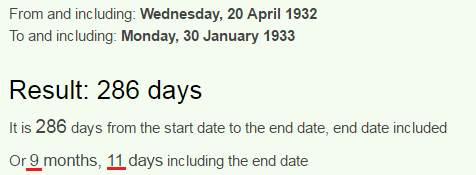Also from January 30th to his 44th birthday is 2 months and 22 days, which is also 11 weeks and 4 days (His birthday in 1933 has 22 numerology as well - 4/20/1933 = 4+2+0+1+9+3+3 = 22)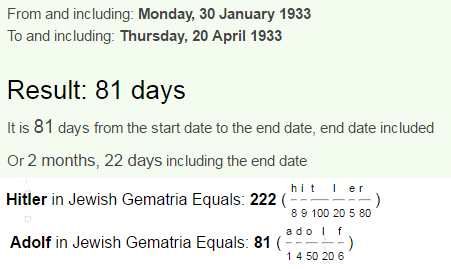Nazis = 33, Holocaust = 33, Berlin = 33, Zyklon B = 33 (Gas used to kill people in concentration camps)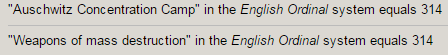And from his birthday in 1934 to the day when The 2nd President of Germany died and he became Fuhrer is 3 months and 14 days  ( 8/2/34 - 8+2+34 = 44)Masonry - 105, Zionism - 105
In numerology 105 is seen as 15 since 0 doesn't count, 15th prime number is 47, also 105 days is exactly 15 weeks

From his birthday in 1934 to his death - 4/30/1945 is exactly 11 years and 11 moths...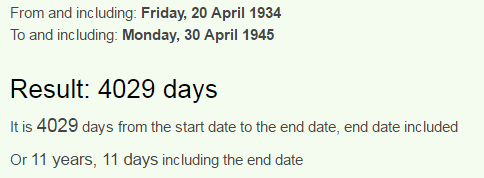Moving on from measuring days for nowAlso known as Nazi Party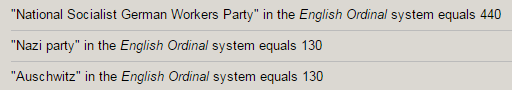Hitler was chairman of Nazi party for almost entirety of it's existence

It was founded on February 24th in 1920 - February is the only month that sums to 42, it was established on 24th day a reflection of 42 (Hitler was chairman for 24 years)
February 24th is 55th day in the year
World War Two = 5+6+9+3+4+ 5+1+9 + 2+5+ 6 = 55

2/24/1920 - 2+24+1+9+2+0 = 38 (Germany = 38, Death = 38, Killing = 38, Adolf = 38, Jew = 38, Palestine = 38, Soldiers = 38)

Schutzstaffel  (SS) was a military order created 5 years after establishment of Nazi Party
SS flag can be seen as 44 (Kill = 44, Military = 44, Genocide = 44.. )
If you take it as double SS it can be 38 (Death = 38, Killing = 38, Soldiers = 38, Germany = 38, Adolf = 38)
Formed on April 4th, 1925 (4/4 a lot like 44), April Fourth leaves 271 days in the year (58th prime number is 271 - Freemasonry = 58, Secret Society = 58, Solomon's Temple = 58 Global economy = 58) also the Agency was dissolved on May 8th which can be written as 5/8

Formed - 4/4/25 = 4+4+25 = 33
Dissolved - 5/8/45 = 5+8+45 = 58
5/8/1945 - 5+8+19+45 = 77 (33-77 is very frequent) Judaism = 77, Christ = 77

They also say that SS was officially founded on 11/9/1925

11/9/1925 - 1+1+9+1+9+2+5 = 28 (Twenty-eight = 156, Thirty-three = 156, 156th prime number is 911 a reflection of 119)

119 has a connection to 144, but is a popular number on itself being a reflection of 911 and Star of David = 119, All seeing eye = 119, Foundation = 119, Saturn = 119 (In Francis Bacon system - lower case A-Z is 1-26, upper case A-Z is 27-52)

5.5 million - 6 million Jews is truly hilarious to me
But they can give us even more
44th prime number is 193..

Also I forgot to mention that Hitler's wife Eva Braun was 33 years old at the time they committed suicide